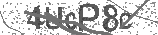﻿ The 2 Times Table Song (Multiply by 2) | Silly School Songs | Safe Videos for Kids
Welcome

# The 2 Times Table Song (Multiply by 2) | Silly School Songs

Thanks! Share it with your friends!

URL

You disliked this video. Thanks for the feedback!

Sorry, only registred users can create playlists.URLChannel: Silly School Songs
Categories: Basic Math   |   MathFind Related Videos  added
120 Views## Description

Learn how to multiply by 2 with this upbeat and catchy song! For more music and information, visit http://sillyschoolsongs.com.

FREE Practice and Speed Drill for the two times table!!
http://sillyschoolsongs.com/multiply-by-two-free-speed-drill-and-practice/

Lyrics:

Get ready class for your 2 times table
Multiply by 2, we know you're able
Get ready class for your 2 times table
Multiply by 2, we know you're able
(Let's go!)

2 x 1 = 2
2 x 2 = 4
2 x 3 = 6
2 x 4 = 8
2 x 5 = 10
2 x 6 = 12
2 x 7 = 14
2 x 8 = 16
2 x 9 = 18
2 x 10 = 20
2 x 11 = 22
2 x 12 = 24

Multiplication is repeated addition
Let's turn the numbers around in the opposite position
Starting with 1 we'll go up to 12
Say it with us 'til you can do it yourself

1 x 2 = 2
2 x 2 = 4
3 x 2 = 6
4 x 2 = 8
5 x 2 = 10
6 x 2 = 12
7 x 2 = 14
8 x 2 = 16
9 x 2 = 18
10 x 2 = 20
11 x 2 = 22
12 x 2 = 24

Bye Bye!!!

Copyright 2015 Silly School Songs. All Rights Reserved.## Post your comment## Comments

Be the first to comment